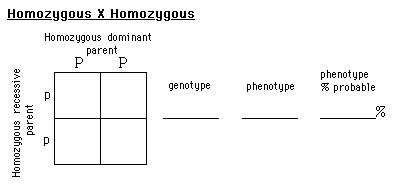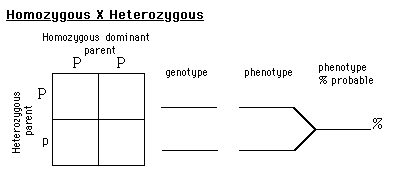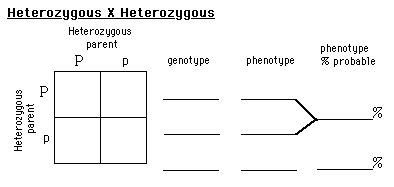Monohybrid Corn Lab

 Monohybrid Cross in CornINTRODUCTION:

A cross between individuals that involves one pair of contrasting traits is called a monohybrid cross. First we will use Punnett square diagrams to predict the results of various monohybrid crosses. We will then examine ears of corn Purple results from the dominant allele (P), and yellow from the recessive allele (p). We will be making observations and assumptions for both the genotype or genetic make-up, and the phenotype or external appearance.
Review genetics and the use of Punnett squares in a biology text before doing this experiment.

MATERIALS:

Appropriate ears of corn.
(You can purchase them from a biological supply, such as Carolina. You need a heterozygous X heterozygous 3:1, and a monohybrid test cross 1:1.)

PROCEDURE:

Theoretical: We will use a Punnett square to examine the theoretical outcome of possible monohybrid crosses.

1. The first cross is with a Homozygous dominant parent (PP), and a Homozygous recessive parent (pp).
Fill in the Punnett square. Each box represents a genotype possibility for an offspring. Place the allele donated by each parent in the corresponding box. Now list the possible genotypes and their corresponding phenotype.
Remember: The genotype is represented by the two letters for the offspring, and the phenotype is a color.
Remember: If an individual’s genotype is heterozygous, the dominant trait will be expressed in the phenotype.
Give the percent possible for the phenotypes.2. Now look at a cross between a Homozygous dominant parent (PP), and a Heterozygous parent (Pp). Fill in as in step one.3. Next we will examine the possibilities with a cross between a Heterozygous parent (Pp), and another Heterozygous parent (Pp). Fill-in as before.4. Finally we will examine a Test cross. A test cross is between a Homozygous recessive parent (pp), and a Heterozygous parent (Pp). Again, fill-in as before.Actual cross: Now we will make a count of an actual cross and compare the calculations to the phenotype percentages of the theoretical.

5. Obtain an ear of corn that is the result of Heterozygous X Heterozygous. Count and record the purple and yellow kernels, and record the numbers. Also record the total number of kernels.

TOTAL purple: _______ TOTAL yellow: _______ TOTAL all kernels: _______

6. Now find the percent of purple and yellow kernels. To find the percent of purple, divide the total purple by the total for all kernels, then multiply by 100.

Percent purple: _______ % Percent yellow: _______ %

Compare your results with the theoretical answers you obtained for the Heterozygous X Heterozygous cross.

7. Results for genetic crosses are often recorded as ratios. Calculate the ratio of purple to yellow. To do this, use their totals. The smaller total you call 1, and write it in the appropriate space. Then Divide the larger one by the smaller, and round to the nearest whole number. Record this number in the appropriate space.

Ratio of phenotypes for this cross.

PURPLEtoYELLOW
____:____

8. Now repeat steps 5 through 7 for an ear of corn that is the result of a TEST CROSS: Homozygous recessive X Heterozygous.

TOTAL purple: _______ TOTAL yellow: _______ TOTAL all kernels: _______

Percent purple: _______ % Percent yellow: _______ %

Compare your results with the theoretical answers you obtained for the Test cross.

Ratio of phenotypes for this cross.

PURPLEtoYELLOW
____:____